Orginal Article

# 基于气液相界面捕捉的统一气体动理学格式

1西北工业大学动力与能源学院, 西安 710072
2西北工业大学陕西省航空发动机内流动力学重点实验室, 西安 710072
3先进航空发动机协同创新中心, 北京 100191;

# UNIFIED GAS-KINETIC SCHEME FOR TWO PHASE INTERFACE CAPTURING

Wang Zhao1, Yan Hong123*1School of Power and Energy, Northwestern Polytechnical University, Xi’an 710072, China;
2Shaanxi Key Laboratory of Internal Aerodynamics in Aero-Engine, Northwestern Polytechnical University, Xi’an 710072, China;
3Collaborative Innovation Center for Advanced Aero-Engine, Beijing 100191, China;

Abstract

The study of interfacial motion of gas-liquid phase is very important in science and engineering. Considering the non-equilibrium flow calculation, a unified gas-kinetic scheme for gas-liquid two phase interface capturing is presented in this paper. Since the free transport and particle collision are coupled to update the macroscopic variables and microscopic distribution functions, the unified gas-kinetic scheme can solve the non-equilibrium flow. The van der Waals (vdW) equation of state (EOS) is included to describe the coexistence of gas and liquid and the phase transition between them. Because of the characteristics of vdW EOS, the interface between gas and liquid can be captured naturally through condensation and evaporation processes. As a result, the new scheme can solve the gas-liquid two phase problems. Finally, the proposed method is used to obtain the numerical solution of Maxwell construction, which agrees well with the corresponding theoretical solution. Then, the Laplace’s theorem is verified by numerical calculation of the surface tension of the droplet corresponding to the van der Waals state equation. In addition, the collision of the two droplets is simulated, which proves the validity of the scheme further. However, due to the characteristics of the van der Waals equation of state, the constructed scheme is only applicable to the case where the liquid/gas two-phase density ratio is less than 5.

Keywords： unified gas-kinetic scheme ; van der Waals equation of state ; interface capturing ; Maxwell construction ; surface tension

0

Wang Zhao, Yan Hong. UNIFIED GAS-KINETIC SCHEME FOR TWO PHASE INTERFACE CAPTURING[J]. Acta Mechanica Sinica, 2018, 50(4): 711-721 https://doi.org/10.6052/0459-1879-17-364

## 1 问题描述

$p=8ρT3-ρ-3ρ2$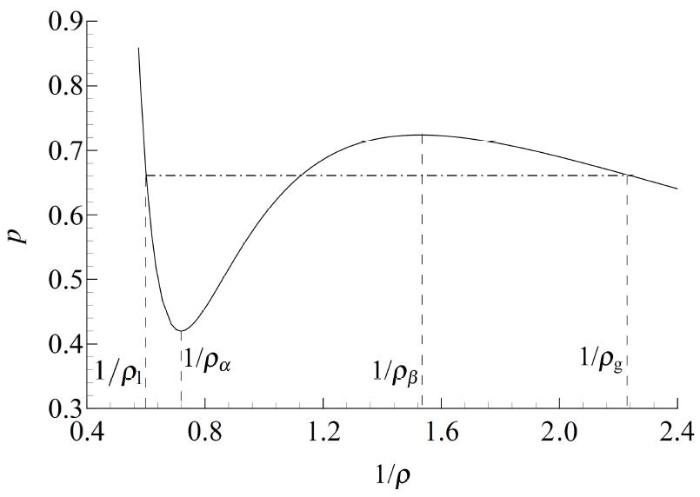Fig.1   The schematic diagram for van der Waals equation of state.

## 2 基于气液相界面捕捉的统一气体动理学格式

### 2.1 动理学模型

$ft+ufx+vfy=g-fτ$

$λ=ρ2p=3-ρ6ρ2-18ρ+16T=Λ(ρ)$

### 2.2 简化动理学模型

$H=∫‍fdξ$

$W=ρρUρV=∫‍HuHvHdudv$

$Ht+uHx+vHy=Heq-Hτ$

### 2.3 基于气液相界面捕捉的统一气体动理学格式

$Hi,jn=Hi,j,α,βn=H(tn,xi,yj,uα,vβ)$

$Wi,jn=∑‍κα,βHi,jnκα,βHi,jnuακα,βHi,jnvβ$

$Hi,j,α,βn+1=Hi,j,α,βn+1Δx∫tntn+1‍fi-1/2,α,βH-fi+1/2,α,βHdt+1Δy∫tntn+1‍gj-1/2,α,βH-gj+1/2,α,βHdt+Δt2⋅Heq,i,j,α,βn+1-Hi,j,α,βn+1τn+1+Heq,i,j,α,βn-Hi,j,α,βnτn$

$Wi,jn+1=Wi,jn+1ΔxFi-1/2,j-Fi+1/2,j+1ΔyGi,j-1/2-Gi,j+1/2$

$Fi-1/2,j=∫tntn+1‍∫‍uψHi-1/2,jdudvdt$$Gi,j-1/2=∫tntn+1‍∫‍vψHi,j-1/2dudvdt$, $ψ=(1,u,v)T$$fi-1/2,α,βH=∫‍uHi-1/2,jdudv,gj-1/2,α,βH=∫‍vHi,j-1/2dudv$首先求解式(8), 得到n+1步的宏观量, 从而得到对应的平衡态, 以此来更新式(7), 得到n+1 步的分布函数. 故而, 发展UGKS 的核心便在于如何构造界面的气体分布函数.

$Ht+uHx=Heq-Hτ$

$H(0,t,u)=1τ∫0t‍Heq(x',t',u')e-(t-t')/τdt'+e-t/τH0(xn,0,un)$

$H0(x,0,uα)=Hi+1/2,αL+σi,αHx,x0Hi+1/2,αR+σi+1,αHx,x>0$

$Hi+1/2,αL=Hi,α+(xi+1/2-xi)σi,αH$

$Hi+1/2,αR=Hi+1,α-(xi+1-xi+1/2)σi+1,αH$

$Heq(x,t,uα)=Heq0[1+(1-H[x])aHLx+H[x]aHRx+AHt]$

$Heq0$

$Mt1=1-e-t/τ$

(1) 将原始的动理学方程(2)变为简化分布函数所对应的方程(9).

(2) 网格界面的简化气体分布函数通过动理学方程的积分解(10)而得到.

(3) 通过式(7)和式(8)分别更新分布函数和宏观量.

(4) 对以上三步进行循环, 便可求得流场的时间演化解.

## 3 算例验证

$pl=Λ(ρl),pr=Λ(ρr)$

### 3.1 麦克斯韦等面积律

$ρini,g,ρini,l$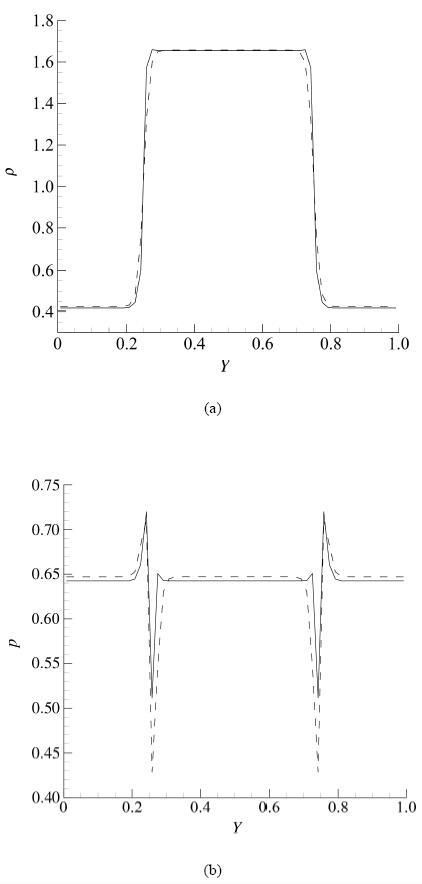Fig.2   Case1: The dotted line is the initial distribution. The solid line is the numerical result of Maxwell Construction.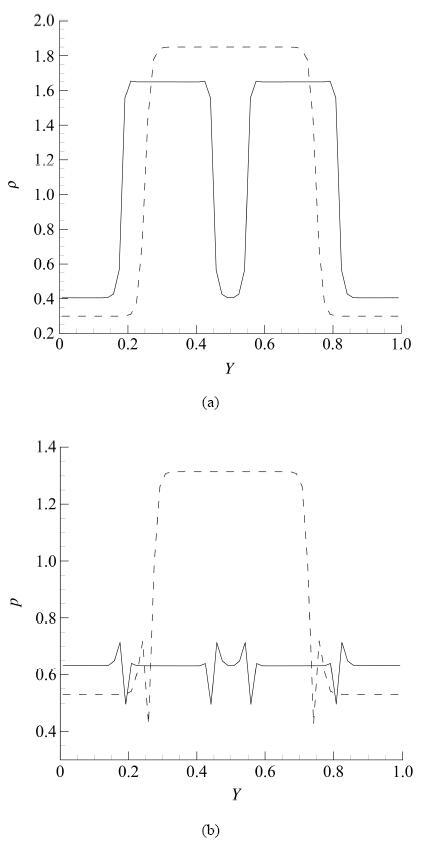Fig.3   Case2: The dotted line is the initial distribution. The solid line is the numerical result of Maxwell Construction.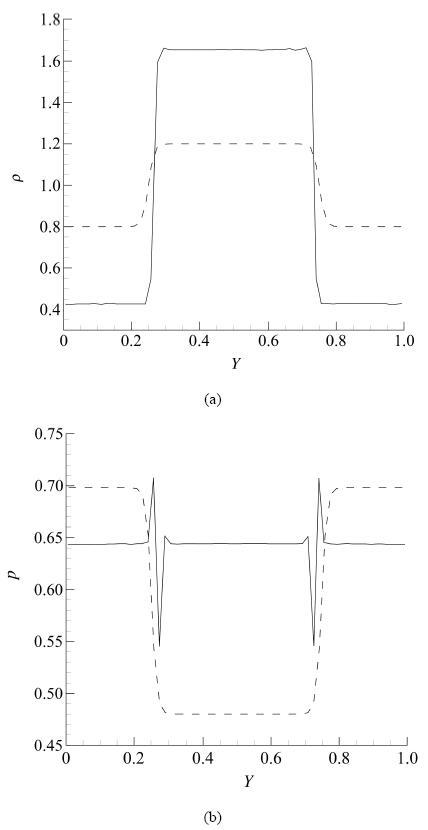Fig.4   Case3: The dotted line is the initial distribution. The solid line is the numerical result of Maxwell Construction.

Table 1   Numerical coexistence solution of different initial values at T = 0.9

CaseInitialCoexistenceRelative
densitiesdensitieserror/%
(gas/liquid)(gas/liquid)
0.425 7/1.657 30.419 4/1.655 01.48/0.14
0.3/1.850.404 4/1.653 75.00/0.22
0.8/1.20.421 3/1.655 91.03/0.08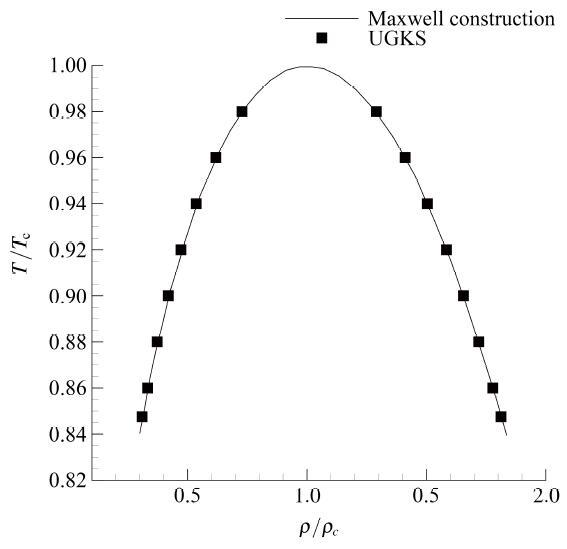Fig.5   Coexistence curve of vdW equation of state.

### 3.2 液滴表面张力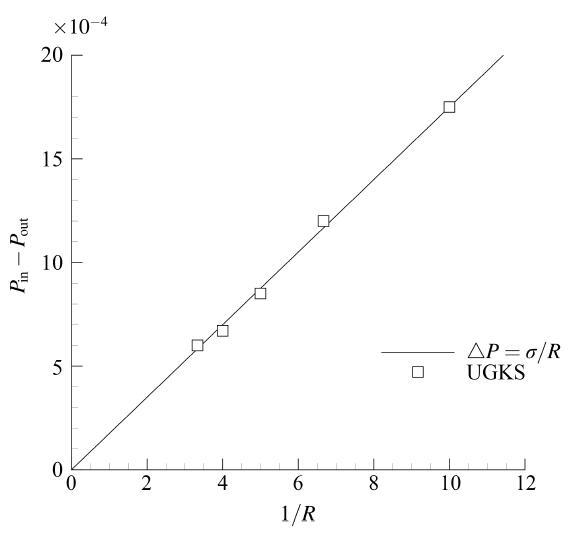Fig.6   The relationship between the pressure difference and the radius of droplet .

### 3.3 液滴碰撞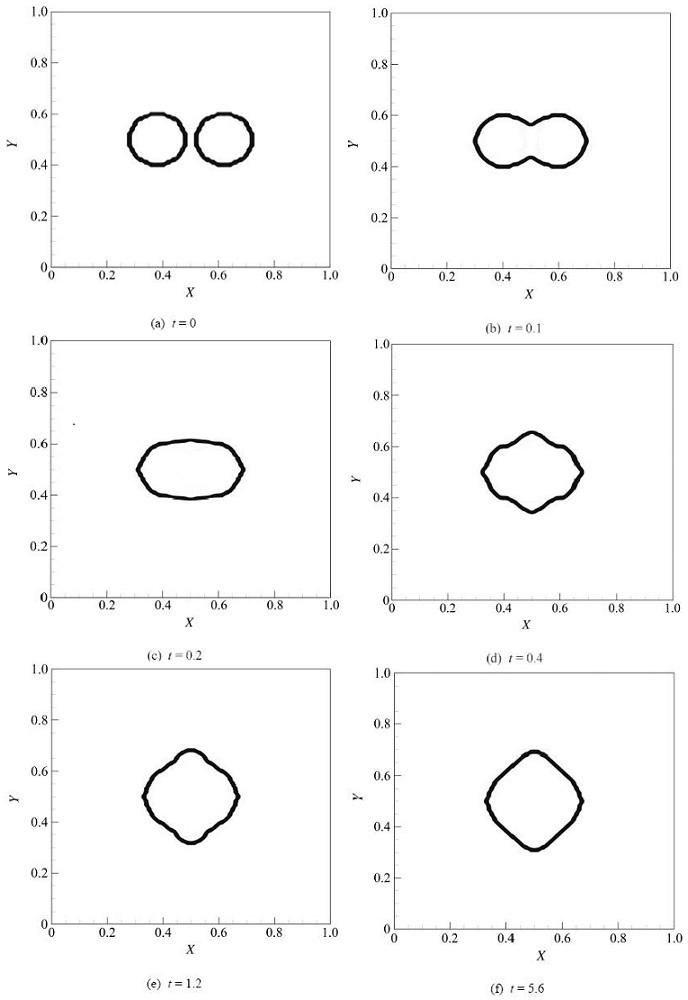Fig.7   Time evolution of the collision of two droplets.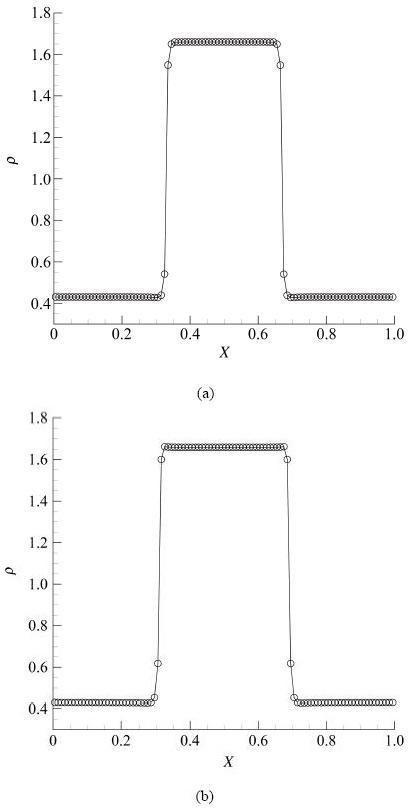Fig.8   The density distribution (a) along the central line of Fig.7 (f) in the X direction and (b) along the central line of Fig.7 (f) in the Y direction.

## 4 结论

The authors have declared that no competing interests exist.

## 参考文献 原文顺序 文献年度倒序 文中引用次数倒序 被引期刊影响因子

  刘文超, 刘曰武. 低渗透煤层气藏中气‒水两相不稳定渗流动态分析. 力学学报, 2017, 49(4): 828-835 (Liu Wenchao, Liu Yuewu.Dynamic analysis on gas-water two-phase unsteady seepage flow in low-permeable coalbed gas reservoirs.Chinese Journal of Theoretical and Applied Mechanics, 2017, 49(4): 828-835 (in Chinese))  李康, 刘娜, 何志伟等. 一种基于双界面函数的界面捕捉方法. 力学学报, 2017, 49(6): 1290-1300 (Li Kang, Liu Na, He Zhiwei, et al.A new interface capturing method based on double interface functions.Chinese Journal of Theoretical and Applied Mechanics, 2017, 49(6): 1290-1300 (in Chinese))  Hirt CW, Nichols BD.Volume of fluid (VOF) method for the dynamics of free boundaries.J Comput Phys, 1981, 39(1): 201-225  张洋, 陈科, 尤云祥等. 壁面约束对裙带气泡动力学的影响. 力学学报, 2017, 49(5): 1050-1058 (Zhang Yang, Chen Ke, You Yunxiang, et al.Confinement effect on the rising dynamics of a skirted bubble.Chinese Journal of Theoretical and Applied Mechanics, 2017, 49(5): 1050-1058 (in Chinese))  Sussman M, Smereka P, Osher S.A level set approach for computing solutions to incompressible two-phase flow.J Comput Phys, 1994, 114(1): 146-159  郭照立, 郑楚光. 格子Boltzmann 方法的原理及应用. 北京: 科学出版社, 2009 (Guo Zhaoli, Zheng Chuguang.Principle and Application of Lattice Boltzmann Method. Beijing: Science Press, 2009 (in Chinese))  Shan X, Chen H.Lattice Boltzmann model for simulating flows with multiple phases and components.Phys Rev E, 1993, 47(3): 1815-1819  Shan X, Chen H.Simulation of nonideal gases and liquid-gas phase transitions by the lattice Boltzmann equation.Phys Rev E, 1994, 49(4): 2941-2948  Gunstensen AK, Rothman DH, aleski SZ, et al. Lattice Boltzmann model of immiscible fluids.Phys Rev A, 1991, 43(8): 4320-4327  Swift MR, Osborn WR, Yeomans JM.Lattice Boltzmann simulation of nonideal fluids.Phys Rev Lett, 1995, 75(5): 830-833  Zhang R, Chen H.Lattice Boltzmann method for simulations of liquid-vapor thermal flows.Physical Review. E, Statistical, Nonlinear, and Soft Matter Physics, 2003, 67(6): 66711  Yuan, P, Schaefer L. Equations of state in a lattice Boltzmann model.Phys Fluids, 2006, 18(4): 42101  Kupershtokh AL, Karpov DI, Medvedev DA, et al.Stochastic models of partial discharge activity in solid and liquid dielectrics.IET Science, Measurement & Technology, 2007, 1(6): 303-311  胡安杰. 多相流动格子Boltzmann 方法研究. [博士论文]. 重庆: 重庆大学, 2015 (Hu Anjie.Study on lattice Boltzmann method for multiphase flow. [PhD Thesis]. Chongqing: Chongqing University, 2015 (in Chinese))  Li Q, Luo KH, Kang QJ, et al.Lattice Boltzmann methods for multiphase flow and phase-change heat transfer.Progress in Energy and Combustion Science, 2016, 52: 62-105  Chikatamarla SS, Karlin IV.Entropic lattice Boltzmann method for multiphase flows.Physical Review Letters, 2015, 114(17): 174502  Wang Y, Shu C, Huang HB, et al.Multiphase lattice Boltzmann flux solver for incompressible multiphase flows with large density ratio.Journal of Computational Physics, 2015, 280: 404-423  Liu H, Kang Q, Leonardi CR, et al.Multiphase lattice Boltzmann simulations for porous media applications.Computational Geosciences, 2016, 20(4): 777-805  Lycett-Brown D, Luo KH.Improved forcing scheme in pseudopotential lattice Boltzmann methods for multiphase flow at arbitrarily high density ratios.Physical Review E, 2015, 91(2): 023305  Shao JY, Shu C.A hybrid phase field multiple relaxation time lattice Boltzmann method for the incompressible multiphase flow with large density contrast.International Journal for Numerical Methods in Fluids, 2015, 77(9): 526-543  郭宇隆. 基于格子Boltzmann 方法的气液混合流体模拟. [硕士论文]. 广州: 华南理工大学, 2015 (Guo Yulong.Study on gas-liquid mixed fluid simulation based on lattice Boltzmann method [Master Thesis]. Guangzhou: South China University of Technology, 2015 (in Chinese))  史冬岩, 王志凯, 张阿漫. 一种模拟气液两相流的格子波尔兹曼改进模型. 力学学报, 2013, 46(2): 224-233 (Shi Dongyan, Wang Zhikai, Zhang Aman.Improved model of simulating gas-liquid two-phase flow by lattice Boltzmann.Chinese Journal of Theoretical and Applied Mechanics, 2013, 46(2): 224-233 (in Chinese))  曾建邦, 李隆键, 廖全等. 格子Boltzmann 方法在相变过程中的应用. 物理学报, 2010, 59(1): 178-185 (Zeng Jianbang, Li Longjian, Liao Quan, et al.Application of lattice Boltzmann method in phase transition process.Acta Physica Sinica, 2010, 59(1): 178-185 (in Chinese))  Gong S, Cheng P.A lattice Boltzmann method for simulation of liquid-vapor phase-change heat transfer.International Journal of Heat and Mass Transfer, 2012, 55(17-18): 4923-4927  Dong L, Gong S, Cheng P.Direct numerical simulations of film boiling heat transfer by a phase-change lattice Boltzmann method.International Communications in Heat and Mass Transfer, 2018, 91: 109-116  Guo Z, Zhao T S, Shi Y.Physical symmetry, spatial accuracy, and relaxation time of the lattice Boltzmann equation for micro gas flows.Journal of Applied Physics, 2006, 99(7): 074903  Zhang Y, Qin R, Emerson DR.Lattice Boltzmann simulation of rarefied gas flows in microchannels.Physical Review E, 2005, 71(4): 047702  Guo Z, Zheng C, Shi B.Lattice Boltzmann equation with multiple effective relaxation times for gaseous microscale flow.Physical Review E, 2008, 77(3): 036707  Shan X, Yuan XF, Chen H.Kinetic theory representation of hydrodynamics: a way beyond the Navier-Stokes equation.Journal of Fluid Mechanics, 2006, 550(1): 413-441  Xu K, Huang JC.A unified gas-kinetic scheme for continuum and rarefied flows.Journal of Computational Physics, 2010, 229(20): 7747-7764  毛枚良, 江定武, 李锦等. 气体动理学统一算法的隐式方法研究. 力学学报, 2015, 47(5): 822-829 (Mao Meiliang, Jiang Dingwu, Li Jin, et al.Study on implicit implementation of the unified gas kinetic scheme.Chinese Journal of Theoretical and Applied Mechanics, 2015, 47(5): 822-829 (in Chinese))  江定武, 毛枚良, 李锦等. 气体动理学统一算法中相容性条件不满足引起的数值误差及其影响研究. 力学学报, 2015, 47(1):163-168 (Jiang Dingwu, Mao Meiliang, Li Jin, et al.Study on the numerical error introduced by dissatisfying the conservation constraint in UGKS and its effects .Chinese Journal of Theoretical and Applied Mechanics, 2015, 47(1): 163-168 (in Chinese))  Xu K.A kinetic method for hyperbolic-elliptic equations and its application in two-phase flow.Journal of Computational Physics, 2001, 166(2): 383-399  Wang Z, Yan H, Li Q, et al.Unified gas-kinetic scheme for diatomic molecular flow with translational, rotational, and vibrational modes.Journal of Computational Physics, 2017, 350: 237-259  Huang H, Sukop M, Lu X.Multiphase Lattice Boltzmann Methods: Theory and Application. Beijing: John Wiley & Sons, 2015  Hu A, Li L, Chen S, et al.On equations of state in pseudo-potential multiphase lattice Boltzmann model with large density ratio.International Journal of Heat and Mass Transfer, 2013, 67: 159-163  Hu A, Li L, Uddin R.Force method in a pseudo-potential lattice Boltzmann model.Journal of Computational Physics, 2015, 294: 78-89  Shan X.Pressure tensor calculation in a class of nonideal gas lattice Boltzmann models.Physical Review E, 2008, 77(6): 066702  强洪夫, 陈福振, 高巍然. 基于SPH 方法的低韦伯数下三维液滴碰撞聚合与反弹数值模拟研究. 工程力学, 2012, 29(2): 21-28 (Qiang Hongfu, Chen Fuzhen, Gao Weiran.Simulation of coalescence and bouncing of three-dimensional droplet collisions with low weber numbers based on SPH method.Journal of Engineering Mechanics, 2012, 29(2): 21-28 (in Chinese))  Nugent S, Posch HA.Liquid drops and surface tension with smoothed particle applied mechanics.Physical Review E, 2000, 62: 4968-4975  Meleán Y, Sigalotti LDG.Coalescence of colliding van der Waals liquid drops.International Journal of Heat and Mass Transfer, 2005, 48: 4041-4061  夏盛勇. 三氧化二铝液滴碰撞机理及模型研究. [博士论文]. 西安: 西北工业大学, 2015 (Xia Shengyong.Physics and model of alumina droplet collisions. [PhD Thesis]. Xi’an: Northwestern Polytechnical University, 2015 (in Chinese))/

 〈〉